# Exporting Your Queries’ M Code From Power Query And Power BI Using Copy/Paste

Two years ago I blogged about a method to export all the M code for all of your queries in Power Query using the Send A Frown button – useful if you need the code for documentation purposes. This trick doesn’t work with Power BI Desktop, unfortunately, but the good news is that there’s a better way to do this now in Power Query and Power BI Desktop using copy/paste. It’s pretty simple really: when you copy a query from the Power Query or Power BI Desktop Query Editor you can not only paste the query to another Query Editor (pasting from Power Query to Power BI and vice versa works too) but you can also paste the query to a text editor like Notepad and get the M code for the query. What’s more, you can also select more than one query in the Query Editor and when you paste you get all the code for all of the selected queries: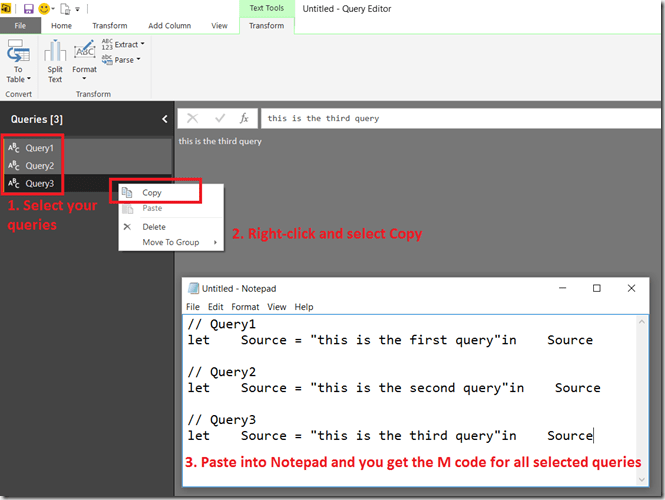Remember that because the properties of each step in a query become comments in your M code, they get copied too.

# Handling Missing Members In The CubeSet() Function With Power Pivot

Last week I received an email from a reader asking how to handle missing members in MDX used in the Excel CubeSet() function. My first thought was that this could be solved easily with the MDXMissingMemberMode connection string property but it turns out this can’t be used with Power Pivot in Excel 2013/6 because you can’t edit the connection string back to the Excel Data Model: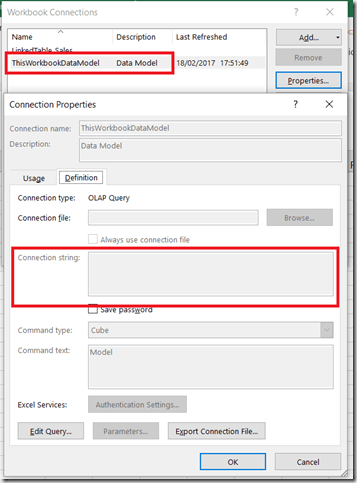Instead, you have no choice but to handle this in MDX.

Here’s an illustration of the problem. Imagine you have the following table of data on your Excel worksheet:With this table added to the Excel Data Model, you could write the following Excel formula using CubeSet():

```=CUBESET(
"ThisWorkbookDataModel",
"{[Sales].[Product].[All].[Apples],
[Sales].[Product].[All].[Oranges],
[Sales].[Product].[All].[Pears]}",
"Set")
```In the screenshot above the CubeSet() formula is used in H3, while in H4 there’s a formula using CubeSetCount() that shows the set contains three members.

If the source data is updated so that the row for Pears is deleted like so:Then the CubeSet() formula returns an error because the member Pears no longer exists:How can this be avoided? If what you actually wanted was all of the Products, whatever they were, the best thing to do is to use the MDX Members function like so:

```=CUBESET(
"ThisWorkbookDataModel",
"{[Sales].[Product].[Product].MEMBERS}",
"Set")
```

[I talk about the Members function in this post in my series of posts on MDX for Power Pivot users]

This formula does not return an error and you can see that the CubeSetCount() formula below shows the set only contains two members now:If you do need to refer to individual members then the MDX you need is more complicated:

```=CUBESET(
"ThisWorkbookDataModel",
"{[Sales].[Product].[All].[Apples],
[Sales].[Product].[All].[Oranges],
iif(
iserror(
strtomember(""[Sales].[Product].[All].[Pears]"")
),
{},
{strtomember(""[Sales].[Product].[All].[Pears]"")}
)
}",
"Set")
```This MDX uses the StrToMember() function to interpret the contents of a string as an MDX expression returning a member; if this expression returns an error then it is trapped by the IsError() function and an empty set is returned.

This isn’t particularly pretty, though, and ideally the MDXMissingMemberMode connection string property would be set to Ignore in the Excel Data Model connection string property.

# Finding Out (Approximately) How Long A Calculation Contributes To The Duration Of An MDX Query

In my last two blog posts (see here and here) I showed how to use the Calculation Evaluation and Calculation Evaluation Detailed Information trace events to work out which MDX calculations are evaluated when a query runs in Analysis Services Multidimensional. That’s very useful, but wouldn’t it be great if you could work out how long any single calculation contributes to the overall duration of a query? If you could, it would make performance tuning MDX calculations much easier.

While you can’t get an exact amount of time taken for each calculation, the good news is that it is possible to get a duration rounded to the next second if your calculation is evaluated in bulk mode.

Take a look at the following query:

```WITH

MEMBER MEASURES.DAYRANK AS
RANK(
[Date].[Date].CURRENTMEMBER,
[Date].[Date].[Date].MEMBERS)-1

IIF(
[Measures].[Internet Sales Amount]=0,
NULL,
MEASURES.DAYRANK)

MEMBER MEASURES.LASTSALERANK AS
MAX(
NULL:[Date].[Date].CURRENTMEMBER,

MEMBER MEASURES.LASTSALE AS
([Measures].[Internet Sales Amount],
[Date].[Date].[Date].MEMBERS.ITEM(MEASURES.LASTSALERANK))

MEMBER MEASURES.SIMPLECALC AS
[Measures].[Internet Sales Amount] * 2

SELECT
*
{MEASURES.SIMPLECALC, MEASURES.LASTSALE}
ON 0,
[Date].[Date].[Date].MEMBERS
ON 1
FROM

```

This query contains five calculated measures: the first four in the WITH clause, DAYRANK, HADSALE, LASTSALERANK and LASTSALE, are based on my approach for finding the last ever non-empty value for a measure across time; the final measure, SIMPLECALC, is as the name suggests a very simple calculation. On my laptop this query takes around 13 seconds to run on my laptop, on a warm Storage Engine cache. Why does it take so long? It’s clearly the calculations that are the problem, but which one(s)?

Luckily all of the calculations in this query are evaluated in bulk mode so, as I discussed in my last two posts, there is an event raised with:

Event Class = Calculation Evaluation Detailed Information

Event Subclass = 107 – RunEvalNode Finished Calculating Item

…for each of the calculations when they are evaluated. Unfortunately the Duration column for this event always shows 0, but there is a way to see approximately how long the calculation took by comparing the Start Time and Current Time columns in the trace.

The 107 – RunEvalNode event for the measure SIMPLECALC shows the same time for the Start Time and Current Time columns: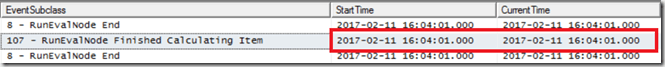This indicates that the SIMPLECALC calculation is evaluated in under a second.

However, sequence of 107 – RunEvalNode events for the LASTSALE calculation shows something different: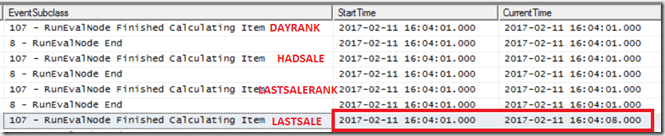There’s a gap of 7 seconds between the StartTime and the CurrentTime, and this indicates that the calculation took 7 seconds to evaluate. It’s a bit frustrating that there isn’t a way to get a more accurate duration here, but it’s still very clear which calculation is taking all the time. Even though the time for calculating LASTSALE includes the time taken for calculating LASTSALERANK, HADSALE and DAYRANK (all of which need to be calculated in order to calculation LASTSALE), the equivalent rows in the trace for these other calculations show they took under a second each. It’s only the logic inside LASTSALE itself that is slow – so that’s where any tuning needs to take place. Indeed, modifying the query to return LASTSALERANK instead of LASTSALE makes the query faster by around 6 seconds, supporting this conclusion.

If you’re curious about what the other 6 seconds of the query execution time is taken up by, it seems like it’s serialisation of the results – something I blogged about here. The query returns a cellset with 400*1190=476000 cells in, and SSAS doesn’t cope well with queries that return a large amount of data.

# Finding Out Which MDX Calculations Are Being Evaluated By Your Query In Analysis Services Multidimensional, Part 2

In part 1 of this series I showed how you can use Profiler to find out which MDX calculations are being evaluated when a query runs on SSAS Multidimensional. In this post I’ll show a practical example of why this is so useful: a situation where SSAS evaluates a calculation that isn’t needed by a query.

Do you have a Date Tool dimension (also known as a Shell dimension or Time Utility dimension) in your cube? A lot of enterprise-level SSAS cubes use this technique to allow you to write a calculation once and have it apply to multiple measures. There are two main approaches to implementing Date Tool dimensions:

• You can create a dimension with one hierarchy and one real member and then use calculated members for your calculations, or
• You can create a dimension with one hierarchy and as many real members as you need calculations, and then use SCOPE statements on these members for your calculations

The second approach, described in detail in this article, is very popular but over the years I have seen several cases where customers of mine who use it have suffered from unexplained query performance problems, problems that have been solved by using the calculated member approach instead. It turns out that the Calculation Evaluation and Calculation Evaluation Detailed Information Profiler events can shed some light on the causes of these problems.

Here’s a simple test cube with a Date Tool dimension that has three real members on it: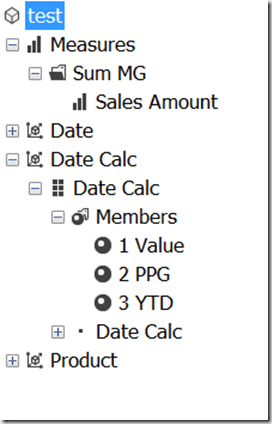Here’s the contents of the MDX Script, copied from the Calculations tab in the Cube Editor in SSDT:

```CALCULATE;

SCOPE([Date Calc].[Date Calc].&[2 PPG]);
THIS = ([Date Calc].[Date Calc].&[1 Value],
[Date].[Calendar].CURRENTMEMBER.PREVMEMBER);
END SCOPE;

SCOPE([Date Calc].[Date Calc].&[3 YTD]);
THIS = AGGREGATE(
YTD([Date].[Calendar].CURRENTMEMBER),
[Date Calc].[Date Calc].&[1 Value]);
END SCOPE;
```

As you can see, two of the members on the [Date Calc] dimension are overwritten by scoped assignments: [2 PPG] is overwritten with a previous period growth calculation and [3 YTD] is overwritten by a year-to-date calculation.

Here’s a query that includes a calculated measure defined in the WITH clause and returns two out of three of the members on the [Date Calc] dimension – but does not return the [3 YTD] calculation:

```WITH
MEMBER [Measures].QueryCalc AS
[Measures].[Sales Amount] + 1

SELECT
{
[Measures].[Sales Amount],
[Measures].QueryCalc
}
*
{
[Date Calc].[Date Calc].&[1 Value],
[Date Calc].[Date Calc].&[2 PPG]
}
ON 0,
[Date].[Calendar].[Month].MEMBERS
ON 1
FROM
TEST
```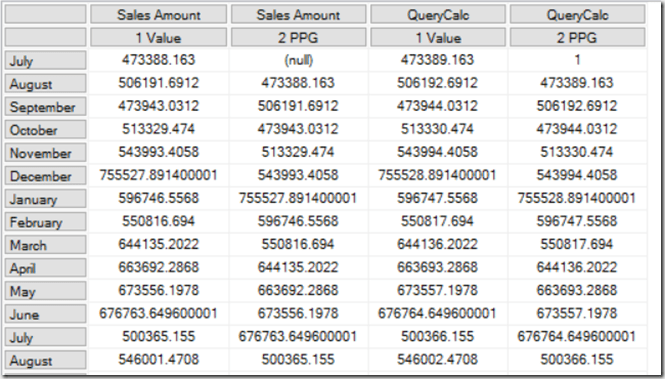Running a Profiler trace as described in my previous post reveals that when this query is run, not only are the [Query Calc] and [2 PPG] calculations evaluated, but [3 YTD] is evaluated too: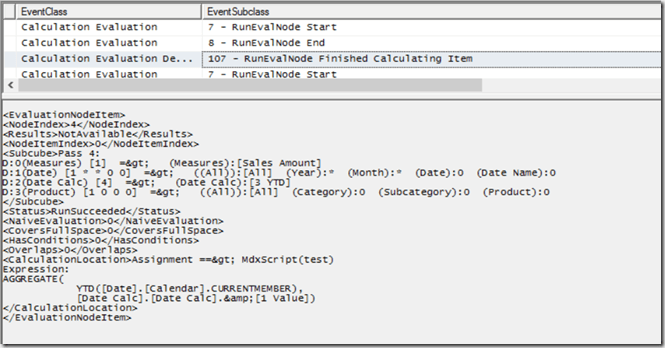It’s worth pointing out that this query was constructed deliberately to show a scenario where SSAS does decide to evaluate the [3 YTD] calculation, but in other cases it may decide otherwise. The reason it decides to do so here is due to a number of factors, including prefetching – see Jeffrey’s blog post here and the section on “Unexpected partition scans” here for some background information on this topic. Remember that in most cases prefetching is a good thing and is beneficial for performance, so if you see something like this happening in your cube you need to be sure that it’s actually causing you a performance problem before you try to prevent it.

If this is a problem for you there are a few things you can do. Rewriting your query to use subselects (if you have control over the MDX query that is being used) is one option:

```WITH
MEMBER [Measures].QueryCalc AS
[Measures].[Sales Amount] + 1

SELECT
{
[Measures].[Sales Amount],
[Measures].QueryCalc
}
*
{
[Date Calc].[Date Calc].MEMBERS
}
ON 0,
[Date].[Calendar].[Month].MEMBERS
ON 1
FROM
(SELECT
{
[Date Calc].[Date Calc].&[1 Value],
[Date Calc].[Date Calc].&[2 PPG]
}
ON 0
FROM
TEST)
CELL PROPERTIES VALUE
```

Using the following connection string properties also works, because it turns off prefetching:

```disable prefetch facts=true; cache ratio=1
```

…but as I said, this might hurt query performance in other ways.

Finally, as I said, using calculated members on your Date Tool dimension instead of the real members/scope statements approach will also work too. In my opinion this is the best solution since the problems with calculated member selection in Excel that caused problems for the calculated member Date Tool approach in the past were fixed a long time ago, and it will work even if you can’t change how your MDX queries are generated.